[BACK]
 Intelligent Automation & Soft ComputingDOI:10.32604/iasc.2020.014192Article

Parallel Equilibrium Optimizer Algorithm and Its Application in Capacitated Vehicle Routing Problem

1College of Computer Science and Engineering, Shandong University of Science and Technology, Qingdao, Shandong, 266590, China
2Faculty of the Built Environment, University of New South Wales, 1466, Australia
*Corresponding Author: JengShyang Pan. Email: jengshyangpan@gmail.com
Received: 04 September 2020; Accepted: 11 October 2020

Abstract: The Equilibrium Optimizer (EO) algorithm is a novel meta-heuristic algorithm based on the strength of physics. To achieve better global search capability, a Parallel Equilibrium Optimizer algorithm, named PEO, is proposed in this paper. PEO is inspired by the idea of parallelism and adopts two different communication strategies between groups to improve EO. The first strategy is used to speed up the convergence rate and the second strategy promotes the algorithm to search for a better solution. These two kinds of communication strategies are used in the early and later iterations of PEO respectively. To check the optimization effect of the proposed PEO algorithm, it is tested on 23 benchmark functions and compared with the Particle Swarm Optimization (PSO), Grey Wolf Optimizer (GWO), Parallel Particle Swarm Optimization (PPSO), and EO as well. The empirical study demonstrates that the abilities of exploration and exploitation of PEO are superior to the above four algorithms in most benchmark functions. Finally, we apply PEO to solve the Capacitated Vehicle Routing Problem (CVRP) in the field of transportation. Experimental results show that PEO can achieve a better driving route.

Keywords: Equilibrium optimizer; parallelism; communication strategy; capacitated vehicle routing problem

1  Introduction

Traditional optimization algorithms usually show better performance in solving single-extreme value optimization problems. But in fact, the practical engineering problems are multiple, extreme and complex. The traditional optimization algorithms are difficult to solve these problems. The emergence of intelligent optimization algorithms breaks the above-mentioned bottleneck. When solving complex problems, they can find approximately optimal solutions within an acceptable time and improve efficiency. Therefore, the research of intelligent optimization algorithms is becoming more and more important.

Intelligent optimization algorithms mostly belong to heuristic algorithms. They are mainly used to solve optimization problems, and their inspiration comes from existing phenomena or things [1,2]. As an extension of the heuristic algorithm, the meta-heuristic algorithm given by nature can be roughly split into three categories: swarm intelligence, evolutionary algorithm and physical algorithm. The characteristics of meta-heuristic algorithms based on species behavior are that the optimization process shares the collective information of all individuals. Typical examples are as follows: Cuckoo Search Algorithm (CSA) , Artificial Bee Colony (ABC) algorithm [4,5], Bat Algorithm (BA) , Pigeon-Inspired Optimization (PIO) algorithm , and so on. The meta-heuristic algorithms based on evolution are related to the evolution law of organisms. Among such algorithms, there are the Differential Evolution (DE) [10,11], Symbiotic Organism Search (SOS) [12,13], Quasi-Affine Transformation Evolutionary (QUATRE) , and so on. The DE is an analogy with the early Genetic Algorithm (GA) [17,18]. They are efficient global optimization algorithms with continuous variables and multiple objectives. Finally, the features of meta-heuristic algorithms based on the physical model are that the communication between search agents is described according to the rules in the physical process. The typical algorithms include Simulated Annealing (SA) [19,20], Gravitational Search Algorithm (GSA) , Charged System Search (CSS) , and so on. The Equilibrium Optimizer (EO) algorithm belongs to the meta-heuristic algorithms based on physics, which is a new intelligent optimization algorithm [23,24].

The EO is enlightened by the model that controls the mass-volume balance and adopts a random exploration mechanism. In EO, each particle updates its position through a candidate particle randomly selected from the equilibrium pool, and the adaptive value of control parameters in the algorithm can reduce the moving speed of particles. The abilities of exploration and exploitation are largely due to this random update strategy and the control parameters in the algorithm. And the tests of EO in benchmark functions and engineering problems prove the practicability and effectiveness of the algorithm .

As a new algorithm, the convergence and global search ability of EO need to be further improved. Its balance mechanism leads to the problem of premature convergence. To further advance global search capability and avoid its premature convergence, this paper improves the EO based on parallel ideas. There are many improvement strategies to improve the performance of the meta-heuristic algorithm, like parallel, compact, multi-strategy and space vector improvement . Among them, the parallel strategy is an important algorithm optimization strategy. Parallelized intelligent optimization algorithm expands the original single population into multiple groups, and carries out group communication during the iteration process, which further improves the optimization ability of the algorithm. The most important thing is the communication strategy between groups when designing the parallel algorithm. Up to now, there are many studies on the parallel improvement technology with inter-group communication strategy . For instance, parallel Particle Swarm Optimization (PSO) , parallel Ant Colony Optimization (ACO) [32,33], parallel Cat Swarm Optimization (CSO) , and so on. Compared with the original algorithms, the parallel strategy improves their optimization ability in solving the problem. Inspired by the idea, a parallel EO algorithm, named PEO, is proposed in this paper. In PEO, two different communication strategies are used to ameliorate the exploitation and search ability of the algorithm. The superiority of PEO is proved by the comparison between PEO and several heuristic algorithms on benchmark functions.

To test the ability of PEO in solving practical problems, the Capacitated Vehicle Routing Problem (CVRP) is treated as an application example of PEO. At present, many scholars have done a lot of research in the field of intelligent transportation information . As one of the core issues, the Vehicle Routing Problem (VRP) was first put forward in 1959. Some non-meta-heuristic algorithms, such as the Tabu Search (TS) algorithm [40,41] and Simulated Annealing (SA) algorithm, have been well applied to solve VRP. As the development of meta-heuristic algorithms, like PSO and Sine Cosine Algorithm (SCA) , they have also provided better solutions to VRP. The vehicle routing problem is generally depicted as follows: several cities have different demands for goods, and the distribution center provides vehicles to the city to transport goods. Each time the vehicle departs from the distribution center, it transports the goods to the unallocated cities, and finally returns to the distribution center. As a derivative problem of the VRP, the CVRP needs to meet an important constraint: the load weight of each vehicle cannot exceed the maximum load. The contributions of PEO in CVRP are as follows. Firstly, the parallel improvement strategy improves the exploitation and search abilities of EO. Secondly, as far as we know, EO is applied to CVRP for the first time, which broadens the application field of EO in intelligent transportation.

The rest of this paper is organized as follows. Section 2 gives a brief retrospect to the basic concept of EO. Section 3 formally proposes our PEO algorithm with details. Section 4 evaluates the algorithm using extensive experiments. Further application analysis of the proposed PEO algorithm in the CVRP is demonstrated in Section 5. The last section describes the conclusions about the presented work.

2  Equilibrium Optimizer

In EO, each particle (solution) and its position (concentration) are used as a search agent. During the iteration process, the search agent randomly updates its current position (concentration) according to the equilibrium candidates until the end of the iteration to get the optimal result (equilibrium state). The initialization of EO is similar to the general meta-heuristic algorithm. The initial population consists of randomly distributed particles in the search space, as shown in Eq. (1):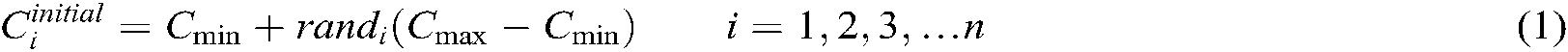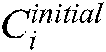is the initial concentration (position) vector of the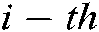particle,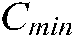is the minimum dimension,is the maximum dimension,is a random vector in the interval of [0,1], andis the whole number of particles.

The EO expects the final equilibrium state to be globally optimum. To heighten the capabilities of exploration and exploitation, an equilibrium pool is established in EO to update the particles. The five particles in the equilibrium pool are called equilibrium candidates. These five particles are the four particles with the best fitness and their average. The four best candidates are beneficial to improve the exploration ability and their average is beneficial to heighten the exploitation ability. Besides, the number of equilibrium candidates is not fixed and can be adjusted for different application problems, which is similar to the selection of three wolves in GWO . The equilibrium pool vector can be expressed as: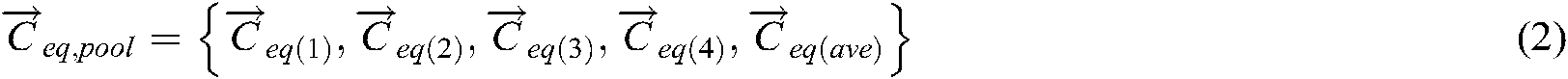To maintain a balance between exploration and exploitation, the parameteris introduced, and the calculation equation is as follows: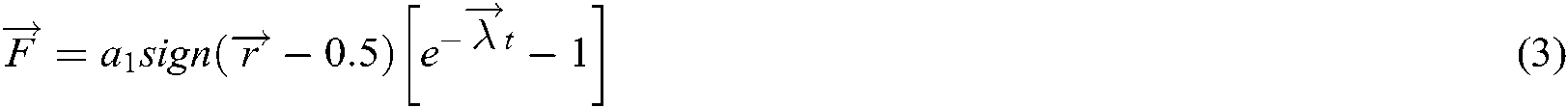In Eq. (3), the calculation equation of the iterative function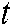is as follows: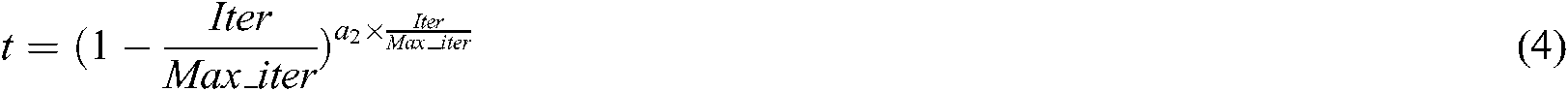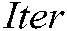means the current number of iterations,is the maximum number of iterations, and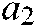is a constant with a value of 1.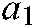is equal to 2. It should be noted that the values ofandare not fixed to 2 and 1, which can be adjusted according to the problem to be solved.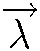andare random vectors in the interval of [0,1].

The parameteris introduced into EO to improve the exact solution in the exploitation stage.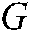is expressed by the following equation: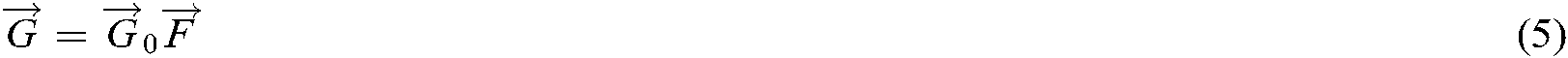where: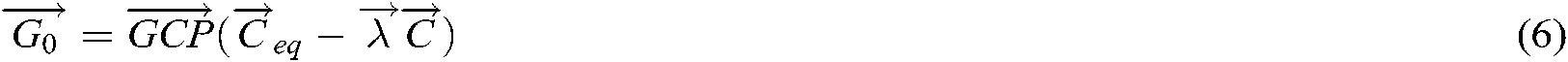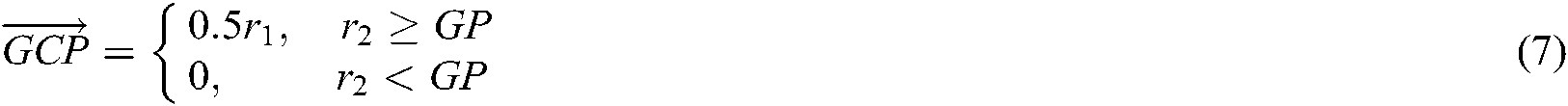In Eq. (7),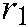andare two random numbers in [0,1], and the parameter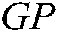is the generation probability. Experiments show that when the value ofis 0.5, the algorithm can keep a good balance between exploration and exploitation. Finally, the update equation of EO is as follows: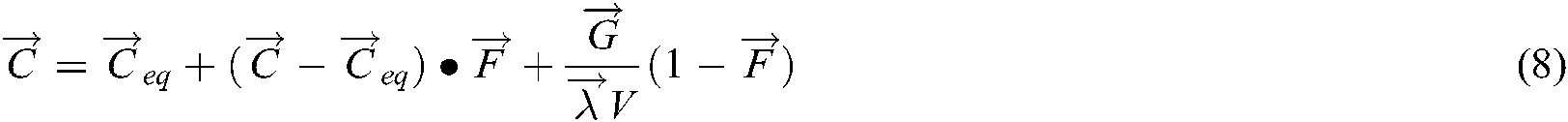In the Eq. (8),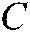represents the particle to be updated. The first part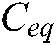on the right side of the equation is an equilibrium candidate particle obtained in the equilibrium pool. The second and third terms represent a change in the concentration of particles in the population. The second term attempts to find an optimal solution in the solution space, and the third term helps to make the solution more accurate. During the iteration process, each particle is updated through an updated equation to improve the adaptability of the particle and the overall optimization ability of the algorithm.

3  Parallel Equilibrium Optimizer

In this section, we propose a parallel Equilibrium Optimizer algorithm, named PEO, to improve the optimization capability of EO. In PEO, a multi-group structure is adopted, and each group search strategy is consistent with the original EO. Regularly, particles from different groups communicate with each other to increase cooperation among groups. Two communication strategies are used in PEO. The first strategy of inter-group communication is used for individual mutation of poor particles, which can heighten the convergence speed of the algorithm. The second strategy is to replace the poor particles in each group, which can ameliorate the exploitation capacity of the algorithm. The two strategies are respectively applied in the early and late stages of the algorithm to further improve the convergence results. Besides, the, as an important parameter, affects the exploitation capability of the algorithm. Therefore, the parameteris micro adjusted according to the number of iterations to better heighten the search capacity of the algorithm in the exploitation stage on the parallel mechanism. The evaluation equation ofis as follows: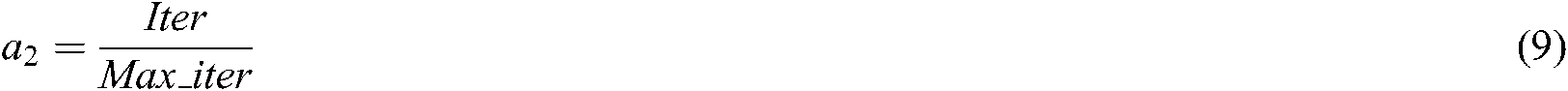In the initialization phase, the groups are divided according to the predetermined total number of particles. In each group, the fitness values of all particles are calculated and sorted, to get the optimal solution and four equilibrium candidates in the group. Comparing all groups, we can get the global optimal solution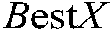of the entire population and four global optimal equilibrium candidates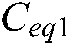,,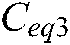, and. After that, each group evolves independently. When a certain number of iterations are performed, the above two strategies will be executed to communicate between groups. Concerning the PEO of this paper, the number of groups is set to 6, and the number of communication iteration intervals is set to 20.

The first inter-group communication strategy is shown in Fig. 1. In the first 1/3 of the total iteration times, a global optimal equilibrium candidate particleis randomly selected and combined with the global optimal particle. According to Eq. (10), individual mutations are performed on the particles with poor fitness in each group. Through this strategy, the mutant particles quickly approach the average position of the equilibrium candidateand the optimal particle, which further improves the convergence speed of the algorithm. The mutation equation is as follow: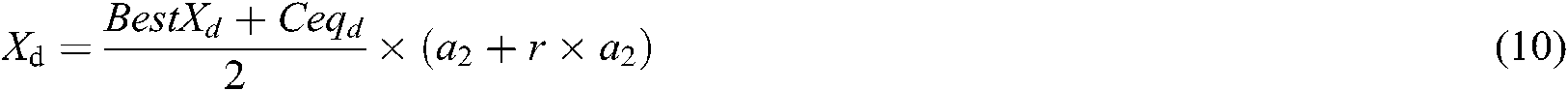whereandare the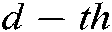dimensions of the mutated particle and global optimal particle.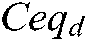represents the d-dimensional position of the equilibrium candidate particle.is a random vector between 0 and 1. The value ofis determined by Eq. (9).

The second inter-group communication strategy is shown in Fig. 2. In the last 2/3 of the whole number of iterations, some particles with poor fitness will be replaced by the average of the optimal particles in this group and the adjacent groups. For example, the average of the optimal particles in the first group and the second group is used to replace the particles with poor fitness in the first group. Repeat this operation until some poor fitness particles in all groups are replaced. This strategy makes the communication between groups more closely, and improves the exploitation ability of the algorithm in the middle and late stages. This operation effectively avoids the premature convergence of the algorithm. In this paper, the number of mutation particles and substitute particles is half of each group. And during the iteration process, the particles are updated according to the structure of EO, regardless of whether component communication has occurred. The particle update equation is shown in Section 2. Figs. 3 and 4 represent the flow chart and pseudo-code of PEO, respectively.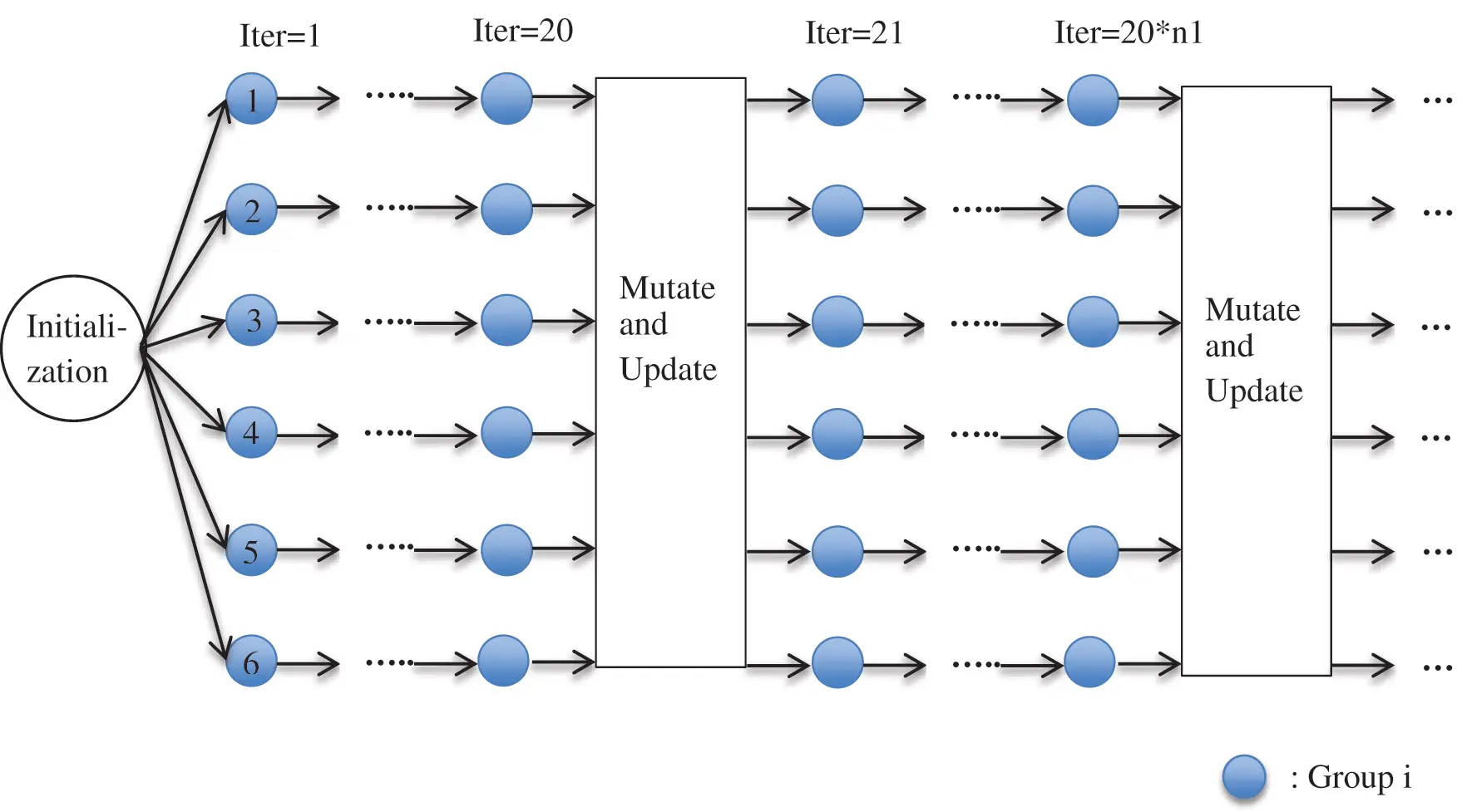Figure 1: Communication strategy 1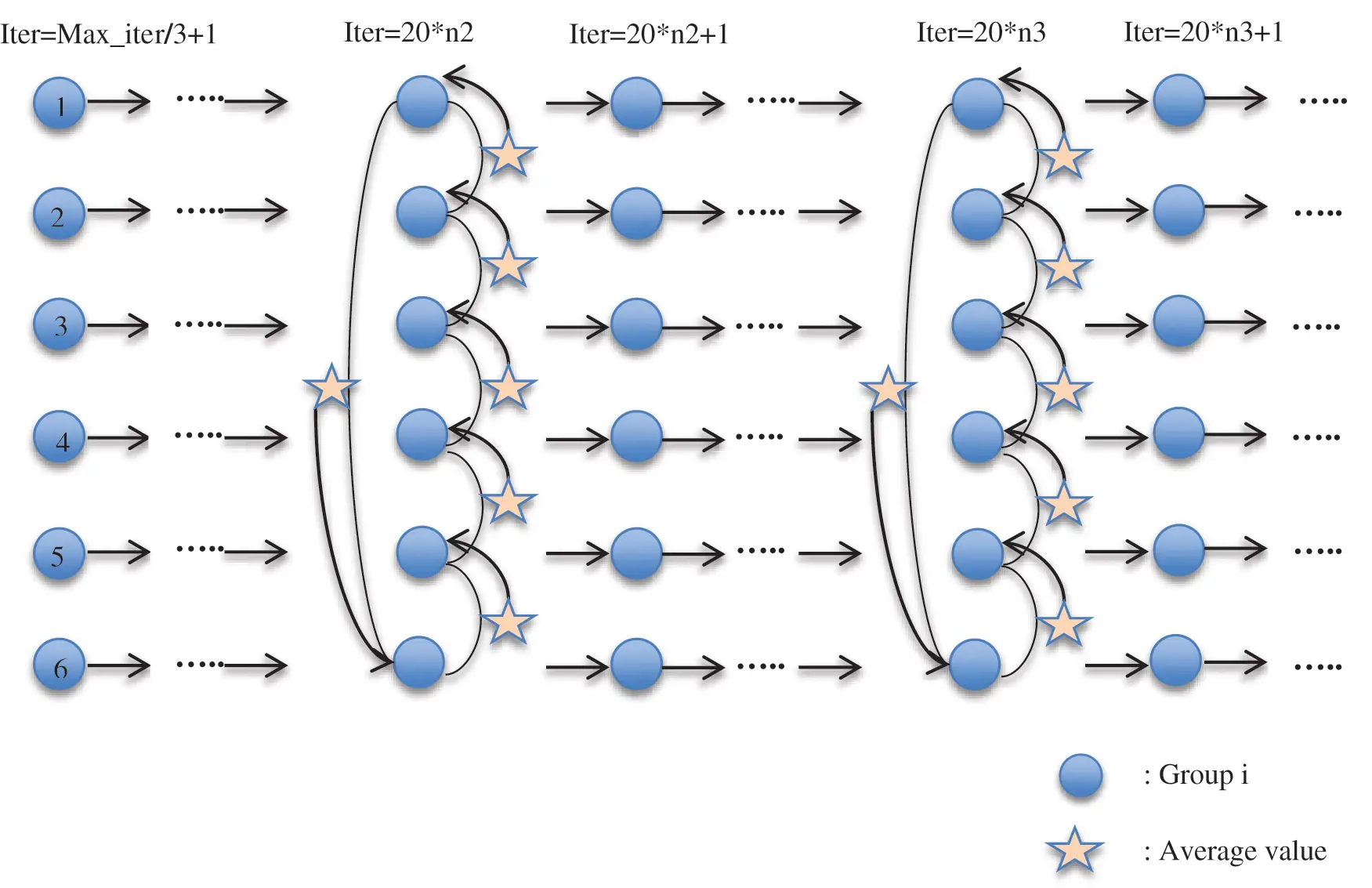Figure 2: Communication strategy 2

4  Results and Discussion

To check the optimization effectiveness of PEO, we test it on 23 benchmark functions and compare it with the PSO, PPSO, GWO, and EO algorithms as well. Among the 23 benchmark functions, F1~F7 represent unimodal functions, F8~F13 represent multimodal functions, and F14~F23 represent fixed-dimensional functions. The detailed description of these functions can be obtained from [21,43]. To make the experiment fair and reasonable, the maximum iteration number of all algorithms is uniformly set as 2000, and the population size is set as 180. Each algorithm is run 10 times independently, and its average (AVG) and standard deviation (STD) are recorded. All simulation experiments are completed in the same experimental environment. The parameter values of the five algorithms are displayed in Tab. 1:

Table 1: The parameter values of five algorithms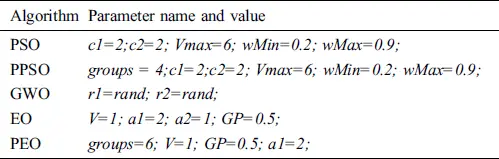Tab. 2 shows the test results of PEO and the other four meta-heuristic algorithms on 23 benchmark functions. The bolded data represents the best average accuracy value of the five algorithms.

Table 2: Results of comparison for the benchmark functions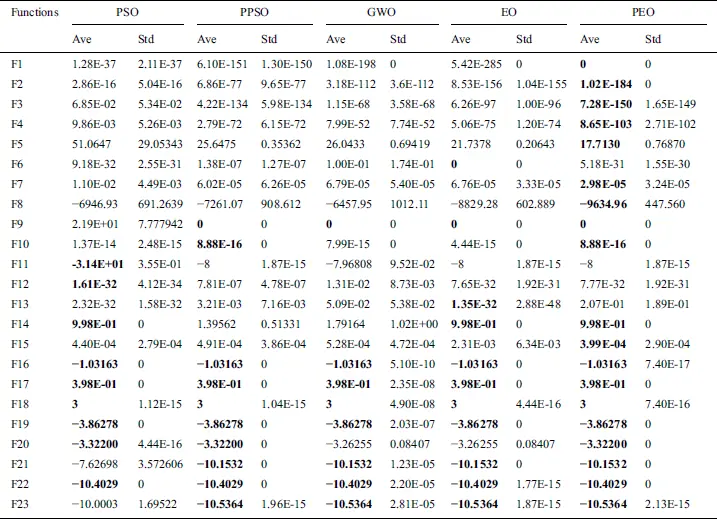It can be known from the bold data in Tab. 2 that PEO can obtain the optimal value of the five algorithms on most benchmark functions. This shows that PEO generally has better optimization ability than PSO, PPSO, GWO, and EO algorithms on the whole. In addition, the standard deviation of PEO is generally small, which indicates that the algorithm has better robustness. For the unimodal functions, PEO displays a better global optimal solution than other algorithms on the functions of F1~F5 and F7, followed by EO. On the function F6, EO performs better. The test results on unimodal functions show that the structure of EO makes its convergence value better than other algorithms. Moreover, the parallel mechanism further improves the convergence effect of EO, which makes PEO perform better on most unimodal functions. For multimodal functions, PEO has a slight advantage over the other four algorithms with respect to optimization ability on functions F8~F10. On functions F11~F13, PSO represents a good convergence effect. But the convergence value of PEO is close to the optimal value of PSO on the function F12. This part of the test data shows that compared with EO, PEO uses the strategy of group communication, which to some extent enhances the ability of the algorithm to jump out of the local optimal solution in multivalued functions. For fixed-dimensional functions F14~F23, PEO can be better than or equal to the convergence results of the other four algorithms. According to the data statistics in Tab. 2, PEO can achieve the best average accuracy on 19 functions, while the corresponding numbers of PSO, PPSO, GWO, and EO algorithms are 9, 10, 8, and 11 in turn. To a certain degree, the results in Tab. 2 also prove the effectiveness of the parallel mechanism in improving algorithm optimization capabilities.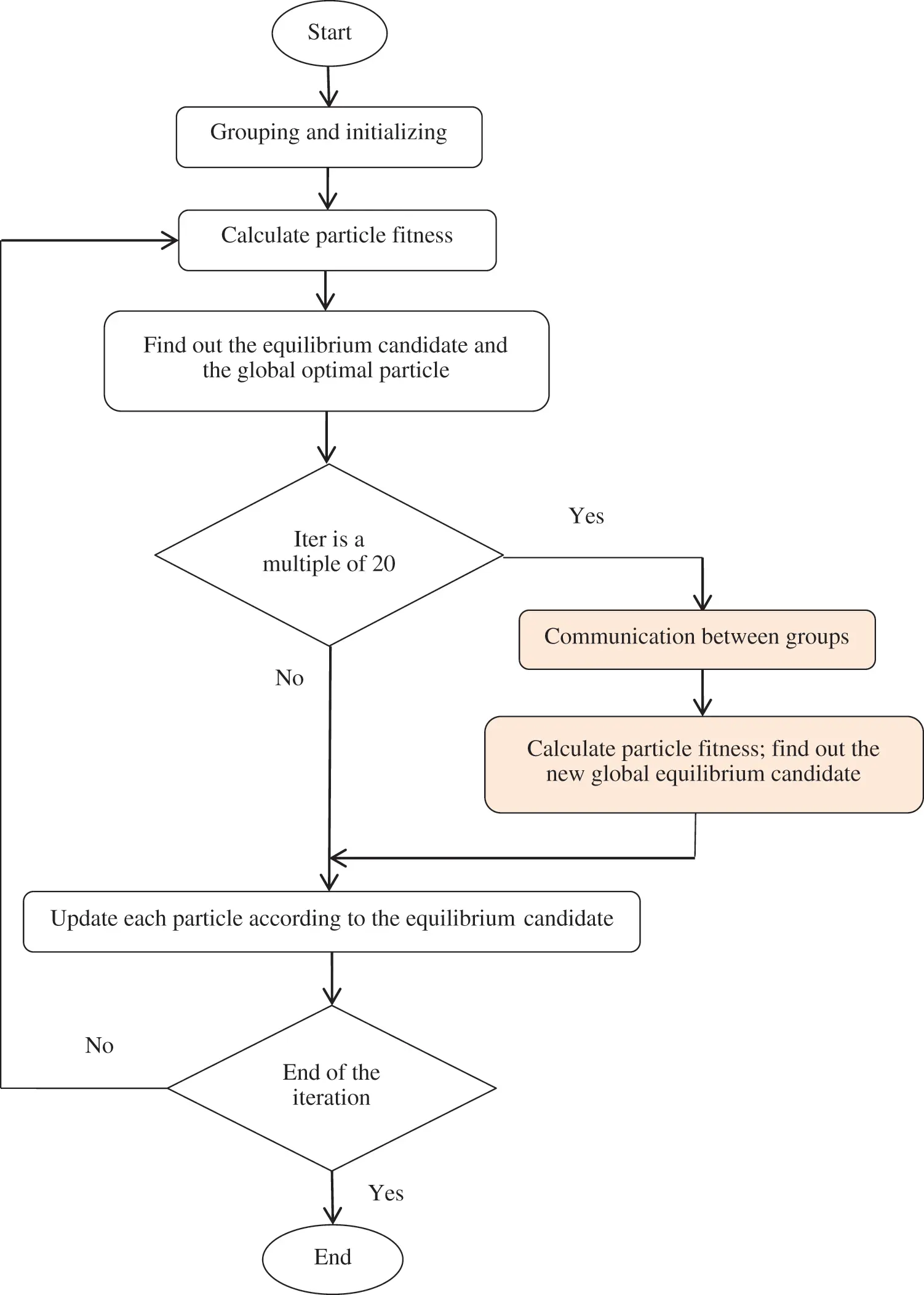Figure 3: Flow chart of parallel equilibrium optimizer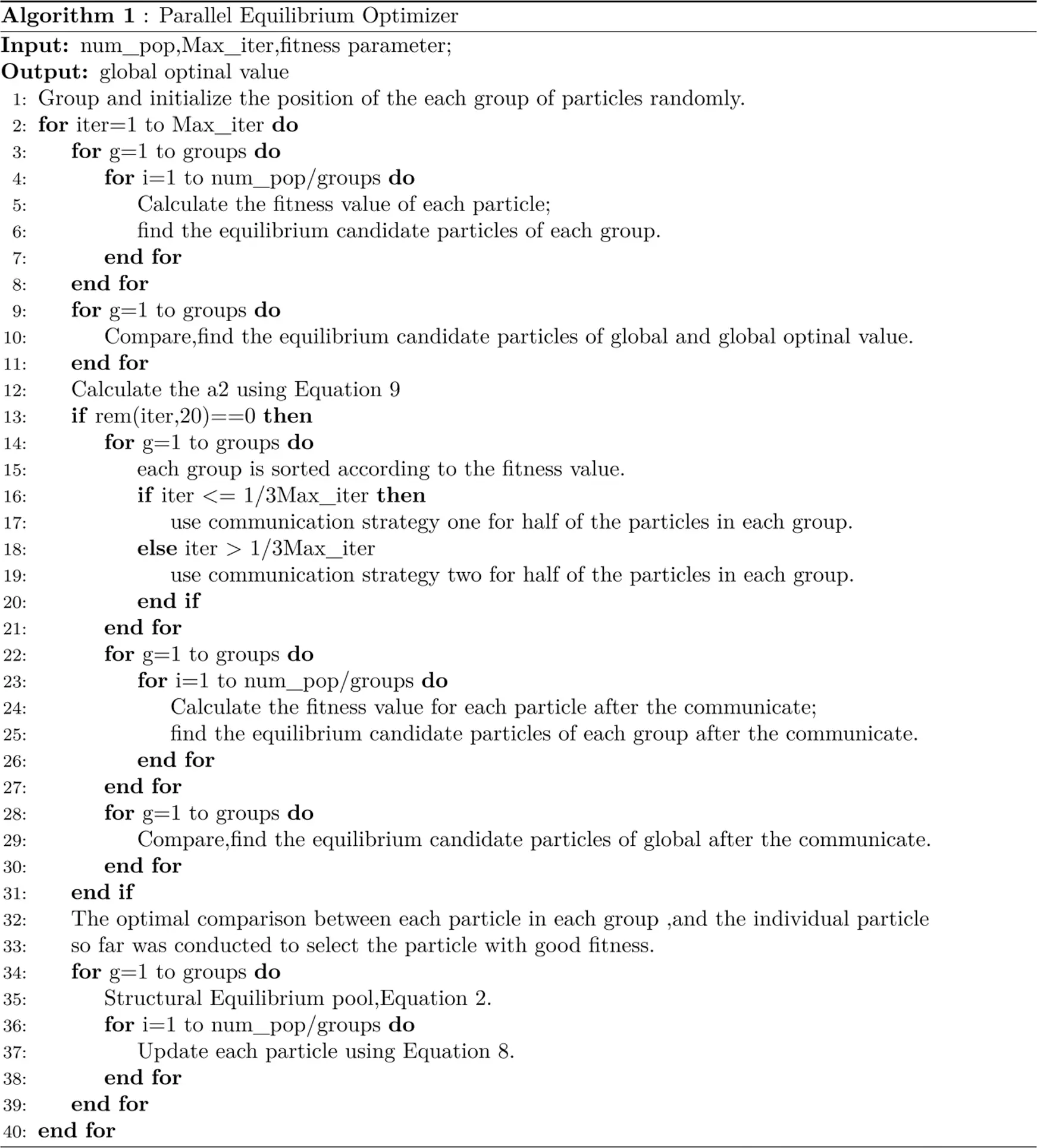Figure 4: Pseudo-code of parallel equilibrium optimizer

To more intuitively observe the experimental results of the five algorithms, 6 groups are selected from 23 groups of experimental graphs to display. Fig. 5 displays the resulting chart of the five algorithms on the unimodal functions F1 and F3, the multimodal functions F8 and F10, and the fixed-dimensional functions F15 and F22. It can be seen from Fig. 5 that compared to other algorithms, PEO has a faster convergence speed. In the result diagram, the test functions image is on the left, and the algorithm convergence curve is on the right. In the algorithm convergence curve, the x-coordinate is the number of iterations, and the y-coordinate represents the functions convergence value.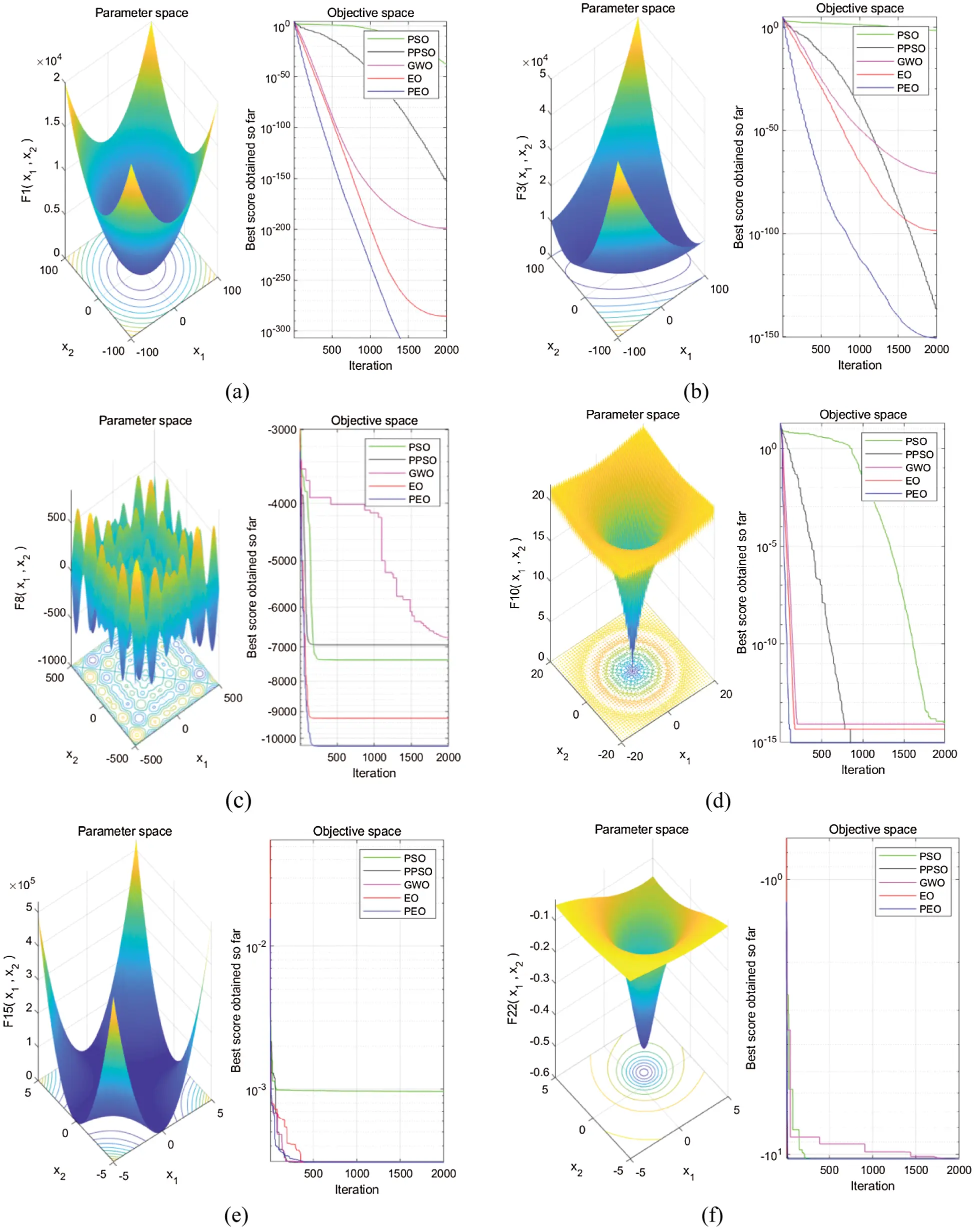Figure 5: Convergence curve of test functions: F1 (a), F3 (b), F8 (c), F10 (d), F15 (e), F22 (f)

5  PEO Algorithm on the Capacitated Vehicle Routing Problem

In this section, we introduce the mathematical model of the Capacitated Vehicle Routing Problem (CVRP) and test the proposed PEO algorithm on the CVRP.

5.1 CVRP Model

In this paper, the parameter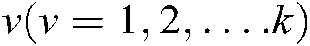is used to represent the vehicle number, and the parameter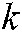represents the total number of vehicles. The distribution center and task city are respectively represented by 0 and. The variables are defined as follows: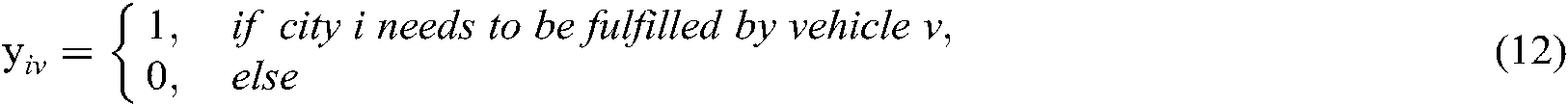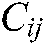means the distance traveled from city pointto city point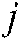,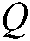represents the maximum load of the car,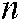is the total number of city points,represents the demand for each city point, the distance traveled of the vehicle is represented by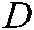, and the solution of the shortest pathis set as the objective function. Finally, the mathematical model of the CVRP can be obtained as shows: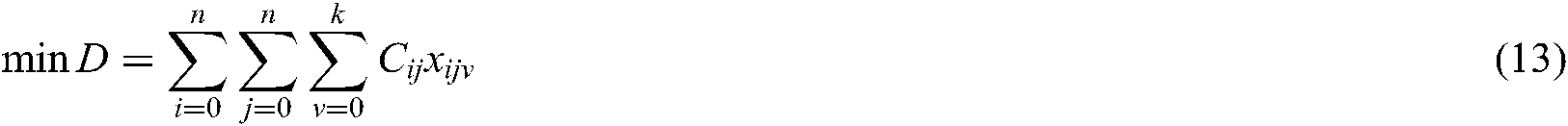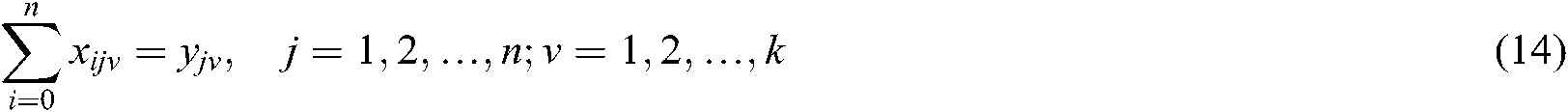In the mathematical model of the CVRP, Eq. (13) means the shortest path of the objective function. Eqs. (14) and (15) ensure that the task of the city point can be completed. Eq. (16) guarantees that each city point can only be completed by one vehicle. Eq. (17) guarantees that the loading weight of each vehicle must not outnumber the maximum loading capacity, which is the most important constraint condition of the CVRP.

5.2 Simulation and Results Analysis

Firstly, a set of simple data is used to test. The task point coordinate and demand are shown in Tab. 3:

Table 3: The task point coordinates and demand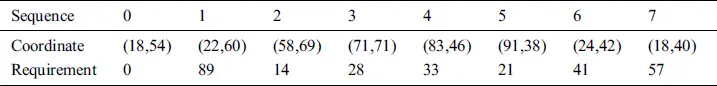In this set of simple test cases, 0 is the central warehouse, the maximum load of the car is set to 100, the task distribution is completed by 3 cars, and the known optimal path is 217.8. In the test, the number of iterations is 200, and the particle swarm size is 180, divided into 6 groups. The test result of PEO reveals in Fig. 6. As can be seen from the Fig. 6, it receives the optimal path 217.8 when PEO iterates to the fifth generation. The results show that PEO has the certain optimization ability in solving VRP.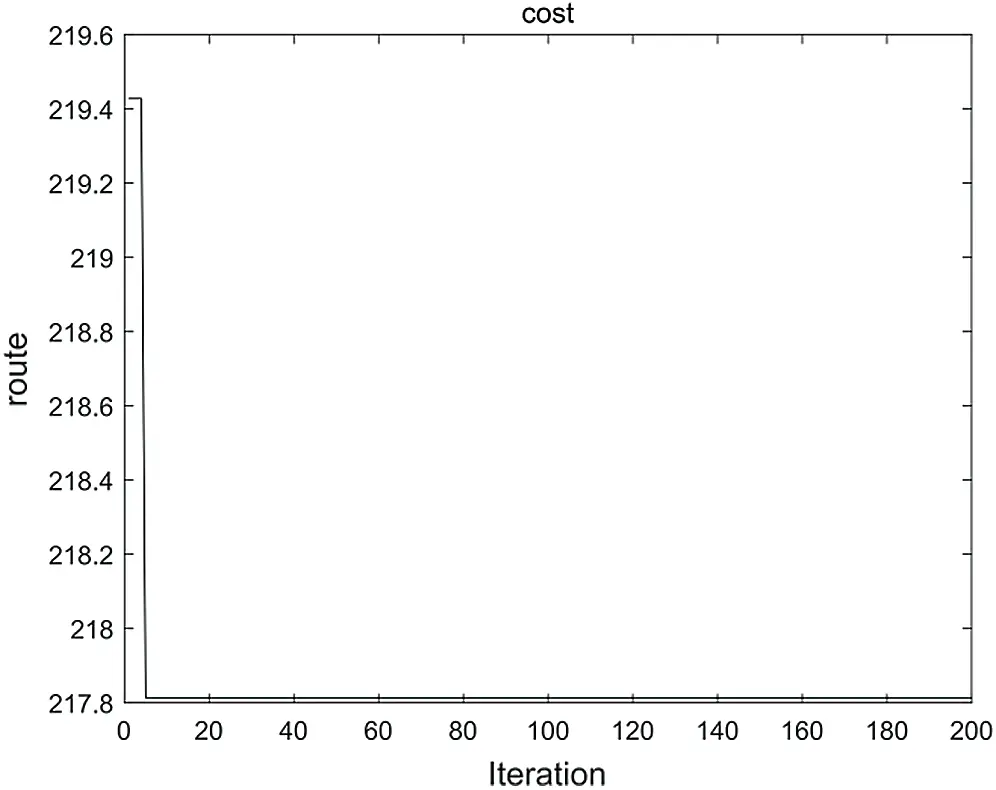Figure 6: The test result of the PEO algorithm under simple test data

To further test the effect of PEO in the CVRP, we select five groups of test data in the CVRP international standard example VRPLIB and compare the results with EO, MMSCA , and PSO algorithms. In this paper, to ensure the fairness of results, the number of iterations for the four algorithms is uniformly set as 3000, and the number of population particles is 180. Tab. 4 records the results of the four algorithms on 5 sets of test data.

Table 4: The result of four algorithms in five test data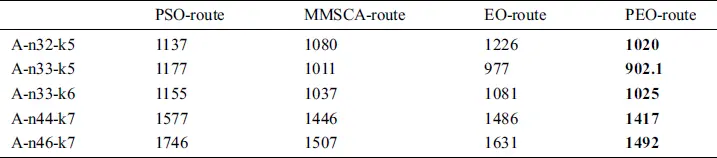Analyzing the data in the Tab. 4, PEO can get a shorter distance than PSO, EO, and MMSCA algorithms on the CVRP under the same experimental conditions. To observe the test results of the four algorithms more intuitively, Fig. 7 shows the change curve of the four algorithms on the CVRP test data A-k32-n5 and A-k33-n6. It can be seen from the Fig. 7 that PEO has a better convergence value than the other three algorithms, yet the convergence speed in the early stage of the iteration is not as fast as other algorithms. Therefore, there is still a lot of room for improvement in the optimization of PEO on the CVRP problem, which can be regarded as the future optimization direction.

6  Conclusion

In this paper, we developed a parallel paradigm for the EO algorithm, called PEO. Two efficient communication strategies are designed and applied to the set of groups in PEO. Experiments show that our approach outperforms existing approaches in most of 23 benchmark functions. Furthermore, applying the PEO algorithm on CVRP can get a shorter distance than PSO, EO, and MMSCA algorithms, which further testify the effectiveness and superiority of the proposed PEO algorithm.Figure 7: Convergence curves of A-k32-n5 (a) and A-k33-n6 (b)

Funding Statement: This work is supported by the National Natural Science Foundation of China [Grant No. 61872085], the Natural Science Foundation of Fujian Province [Grant No.2018J01638], and the Fujian Provincial Department of Science and Technology [Grant No. 2018Y3001]. The author who received the grant is J.S. Pan.

Conflicts of Interest: The authors declare that they have no conflicts of interest to report regarding the present study.

## References

1. J. S. Pan, P. Hu and S. C. Chu. (2019). “Novel parallel heterogeneous meta-heuristic and its communication strategies for the prediction of wind power,” Processes, vol. 7, no. 11, pp. 845.
2. J. Wang, Y. Cao, B. Li, H. J. Kim and S. Lee. (2017). “Particle swarm optimization based clustering algorithm with mobile sink for WSNs,” Future Generation Computer Systems, vol. 76, pp. 452–457.
3. P. C. Song, J. S. Pan and S. C. Chu. (2020). “A parallel compact cuckoo search algorithm for three-dimensional path planning,” Applied Soft Computing, vol. 94, pp. 10644
4. H. Wang, Z. Y. Wu, S. Rahnamayan, H. Sun, Y. Liu et al. (2014). , “Multi-strategy ensemble artificial bee colony algorithm,” Information Sciences, vol. 279, pp. 587–603.
5. P. W. Tsai, M. K. Khan, J. S. Pan and B. Y. Liao. (2014). “Interactive artificial bee colony supported passive continuous authentication system,” IEEE Systems Journal, vol. 8, no. 2, pp. 395–40
6. T. T. Nguyen, J. S. Pan and T. K. Dao. (2019). “A novel improved bat algorithm based on hybrid parallel and compact for balancing an energy consumption problem,” Information-an International Interdisciplinary Journal, vol. 10, no. 6, pp. 194.
7. T. T. Nguyen, J. S. Pan and T. K. Dao. (2019). “A compact bat algorithm for unequal clustering in wireless sensor networks,” Applied Sciences, vol. 9, no. 10, pp. 1973.
8. X. S. Yang. (2010). “A new metaheuristic bat-inspired algorithm,” Nature Inspired Cooperative Strategies for Optimization (NICSO 2010), vol. 284, pp. 65–74.
9. A. Q. Tian, S. C. Chu, J. S. Pan, H. Q. Cui and W. M. Zheng. (2020). “A compact pigeon-inspired optimization for maximum short-term generation mode in cascade hydroelectric power station,” Sustainability, vol. 12, no. 3, pp. 767.
10. X. M. Hu, J. Zhang and H. H. Chen. (2014). “Optimal vaccine distribution strategy for different age groups of population: a differential evolution algorithm approach,” Mathematical Problems in Engineering, vol. 2014, pp. 1–7.
11. A. K. Qin, V. L. Huang and P. N. Suganthan. (2009). “Differential evolution algorithm with strategy adaptation for global numerical optimization,” IEEE Transactions on Evolutionary Computation, vol. 13, no. 2, pp. 398–417.
12. A. E. Ezugwu and D. Prayogo. (2019). “Symbiotic organisms search algorithm: theory, recent advances and applications,” Expert Systems with Applications, vol. 119, pp. 184–209.
13. S. C. Chu, Z. G. Du and J. S. Pan. (2020). “Symbiotic organism search algorithm with multi-group quantum-behavior communication scheme applied in wireless sensor networks,” Applied Sciences, vol. 10, no. 3, pp. 930.
14. Z. Meng, J. S. Pan and H. Xu. (2016). “Quasi-affine transformation evolutionary (QUATRE) algorithm: a cooperative swarm based algorithm for global optimization,” Knowledge-Based Systems, vol. 109, pp. 104–121.
15. Z. Meng and J. S. Pan. (2018). “Quasi-affine transformation evolution with external archive (QUATRE-EARan enhanced structure for differential evolution,” Knowledge-Based Systems, vol. 155, pp. 35–53.
16. Z. G. Du, J. S. Pan, S. C. Chu, H. J. Luo and P. Hu. (2020). “Quasi-affine transformation evolutionary algorithm with communication schemes for application of RSSI in wireless sensor networks,” IEEE Access, vol. 8, pp. 8583–8594.
17. S. W. Mahfoud and D. E. Goldberg. (1995). “Parallel recombinative simulated annealing: a genetic algorithm,” Parallel Computing, vol. 21, no. 1, pp. 1–28.
18. D. Abramson and J. Abela. (1992). “A parallel genetic algorithm for solving the school timetabling problem, ” in Division of Information Technology. Canberra, Australia, 1–11.
19. A. V. Breedam. (1995). “Improvement heuristics for the vehicle routing problem based on simulated annealing,” European Journal of Operational Research, vol. 86, no. 3, pp. 480–490.
20. W. C. Chiang and R. A. Russell. (1996). “Simulated annealing met heuristics for the vehicle routing problem with time windows,” Annals of Operations Research, vol. 63, no. 1, pp. 3–27.
21. E. Rashedi, H. Nezamabadi-pour and S. Saryazdi. (2009). “GSA: a gravitational search algorithm,” Information Sciences, vol. 179, no. 13, pp. 2232–2248.
22. A. Kaveh and S. Talatahari. (2010). “A novel heuristic optimization method: charged system search,” Acta Mechanica, vol. 213, no. 3–4, pp. 267–289.
23. A. Faramarzi, M. Heidarinejad, B. Stephens and S. Mirjalili. (2020). “Equilibrium optimizer: a novel optimization algorithm,” Knowledge-Based Systems, vol. 191, pp. 105190.
24. M. Ahmed, S. Hamdy and K. Salah. (2020). “Extracting model parameters of proton exchange membrane fuel cell using equilibrium optimizer algorithm,” in 2020 IEEE International Youth Conf. on Radio Electronics, Electrical and Power Engineering (REEPERussia, Moscow, pp. 1–7.
25. S. C. Chu, X. S. Xue, J. S. Pan and X. J. Wu. (2020). “Optimizing ontology alignment in vector space,” Journal of Internet Technology, vol. 21, no. 1, pp. 15–22.
26. J. F. Chang, S. C. Chu, J. F. Roddick and J. S. Pan. (2005). “A parallel particle swarm optimization algorithm with communication strategies,” Journal of Information Science and Engineering, vol. 21, no. 4, pp. 809–818.
27. P. W. Tsai, J. S. Pan and S. M. Chen. (2008). “Parallel cat swarm optimization,” in Pro. of the 2008 International Conf. on Machine Learning and Cybernetics, Kunming, China, 6, pp. 3328–3333.
28. X. P. Wang, J. S. Pan and S. C. Chu. (2020). “A parallel multi-verse optimizer for application in multilevel image segmentation,” IEEE Access, vol. 8, pp. 32018–32030.
29. J. Kennedy and R. Eberhart. (2005). “Particle swarm optimization,” in Pro. of ICNN’95-International Conf. on Neural Networks, Perth, Australia, vol. 4, pp. 1942–1948.
30. H. Wang, M. N. Liang, C. L. Sun, G. C. Zhang and L. P. Xie. (2020). “Multiple-strategy learning particle swarm optimization for large-scale optimization problems,” Complex & Intelligent Systems.
31. S. F. Qin, C. L. Sun, G. C. Zhang, X. J. He and Y. Tan. (2020). “A modified particle swarm optimization based on decomposition with different ideal points for many-objective optimization problems,” Complex & Intelligent Systems, vol. 6, no. 2, pp. 263–274.
32. M. Dorigo, V. Maniezzo and A. Colorni. (1996). “Ant system: optimization by a colony of cooperating agents,” IEEE Transactions on Systems, Man and Cybernetics, Part B (Cybernetics), vol. 26, no. 1, pp. 29–41.
33. S. C. Chu, J. F. Roddick and J. S. Pan. (2004). “Ant colony system with communication strategies,” Information Sciences, vol. 167, no. 1, pp. 63–76.
34. S. C. Chu, P. W. Tsai and J. S. Pan. (2006). “Cat swarm optimization,” in 9th Pacific Rim International Conf. on Artificial Intelligence, pp. 854–858.
35. P. W. Tsai, J. S. Pan, S. M. Chen and B. Y. Liao. (2012). “Enhanced parallel cat swarm optimization based on the taguchi method,” Expert Systems with Applications, vol. 39, no. 7, pp. 6309–6319.
36. L. P. Kong, J. S. Pan, P. W. Tsai, S. Vaclav and J. H. Ho. (2015). “A balanced power consumption algorithm based on enhanced parallel cat swarm optimization for wireless sensor network,” International Journal of Distributed Sensor Networks, vol. 11, no. 729680, pp. 1–10.
37. C. L. Lo, H. Y. Kung, C. H. Chen and L. C. Kuo. (2014). “An intelligent slope disaster prediction and monitoring system based on WSN and ANP,” Expert Systems with Applications, vol. 41, no. 10, pp. 4554–4562.
38. C. I. Wu, C. H. Chen, B. Y. Lin and C. C. Lo. (2016). “Traffic information estimation methods from handover events,” Journal of Testing and Evaluation, vol. 44, no. 1, pp. 656–664.
39. C. C. Lo, K. M. Chao, H. Y. Kung, C. H. Chen and M. Chang. (2015). “Information management and applications of intelligent transportation system,” Mathematical Problems in Engineering, vol. 2015, pp. 613940.
40. M. Gendreau, A. Hertz and G. Laporte. (1994). “A tabu search heuristic for the vehicle routing problem,” Management Science, vol. 40, no. 10, pp. 1276–1290.
41. P. Toth and D. Vigo. (2003). “The granular tabu search and its application to the vehicle routing problem,” INFORMS Journal on Computing, vol. 15, no. 4, pp. 333–346.
42. S. Mirjalili. (2016). “SCA: a sine cosine algorithm for solving optimization problems,” Knowledge-Based Systems, vol. 96, pp. 809–818.
43. P. Hu, J. S. Pan and S. C. Chu. (2020). “Improved binary grey wolf optimizer and its application for feature selection,” Knowledge-Based Systems, vol. 195, no. 11, pp. 105746.
44. Q. Y. Yang, S. C. Chu, J. S. Pan and C. M. Chen. (2020). “Sine cosine algorithm with multigroup and multistrategy for solving CVRP,” Mathematical Problems in Engineering, vol. 2020, pp. 1–10.This work is licensed under a Creative Commons Attribution 4.0 International License, which permits unrestricted use, distribution, and reproduction in any medium, provided the original work is properly cited.